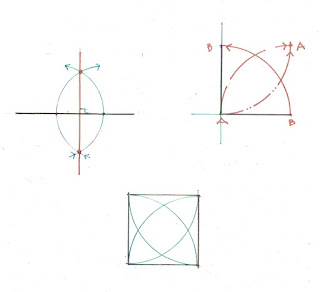## Sunday, December 29, 2019

### Practical Geometry - Drawing a Square with a Compass, Part 1

Draw a square with a compass? !
Yes.
Here are 2 ways. There are several more.Compasses make circles. Straight edges make straight lines. Together they can lay out whatever you can imagine.

How to Draw a Square with a Compass #1

1)   Choose a length: A-B.  It is also the radius: dashed black line A-B,  for drawing a circle with a compass.
2)   Draw the circle.

I have drawn these diagrams on graph paper, a reference to help show how the square grows.

3)  Switch ends. Hold the compass on B. Swing the arc from one side of the circle to the other: G-A-C.
Hold the compass on C. Swing the arc to find D.
Use D to find E; E to find F. along the circumference of the circle.

The circumference of every circle will always be divided into 6 equal parts by the radius of that circle. The length between each 2 points around the circumference will always equal the radius.

It's easy to draw a daisy wheel

However, to construct a square the petals are not needed, only the 6 points on the circumference.

4 )  F-G is the line. It is the same length as the one chosen at the beginning, just in a different location.

G and C are 2 points. that can be connected by a line.
So are F and D.
They are the same distance apart so they are parallel.

A square has 4 equal sides.  (Just a reminder)
5)  An arc the length of  F-G swung from either F or G will mark  G-H and F-I the same length as F-G.  This is the same length as the chosen line AA square drawn using Practical Geometry, using a compass.
To check: lay out the diagonals. If their lengths are equal the square is true.

This upstate NY barn was dismantled for reuse by Green Mountain Timber. It had a daisy wheel scribed on one wall.  The  barn laid out using the 6 points of the circle. The frame of the east elevation is drawn below.The square frame for the door is in the center. Either side completes the rectangle of the circle.

My post describing this barn:

https://www.jgrarchitect.com/2020/03/a-barn-and-its-daisy-wheel.html

How to Draw a Square  with a Compass, #2

Draw a line.  Mark 2 points on the line.
Open the compass wider than the distance between the  points. Swing an arc across the line, below and above it from each point.
The arcs will cross at 2 points. Draw a line between those points. The new line will be perpendicular to the first line.

Then choose the length of the side of the square A-B. Mark it off on both lines.See the arc B-B.
Swing new arcs the same length (A-B)  from both B's.  See the dashed and dash/dotted lines. They cross at both A's.
All the sides are equal: a square.St. Jerome's Catholic Church, East Dorset, VT, 1873, was laid out using that simple square  - including how the arcs cross each other.

My post about it is here:
https://www.jgrarchitect.com/2016/12/st-jerome-catholic-church-east-dorset.html

Part 2 is here:  https://www.jgrarchitect.com/2020/01/practical-geometry-drawing-square-with.html

I explain these ways of using a compass,a straight edge, and a marker to lay out squares and rectangles when I give presentations. I add them here because such information should be readily available on line.